# A 5 Particulate View of Matter What is

• Slides: 19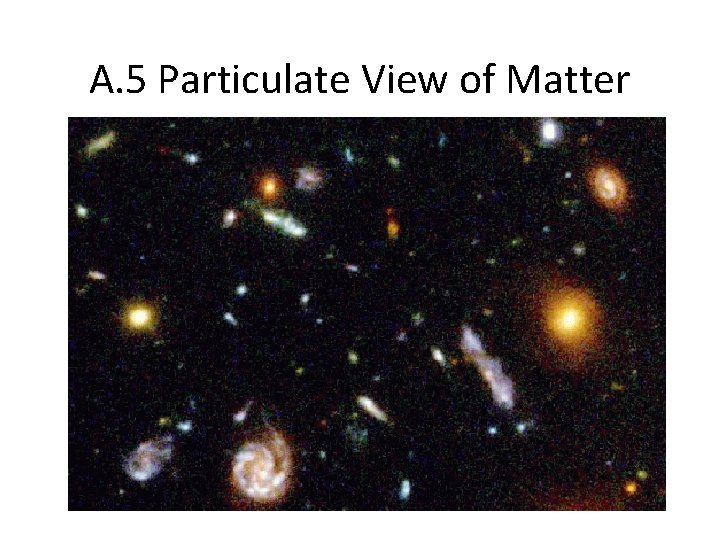A. 5 Particulate View of Matter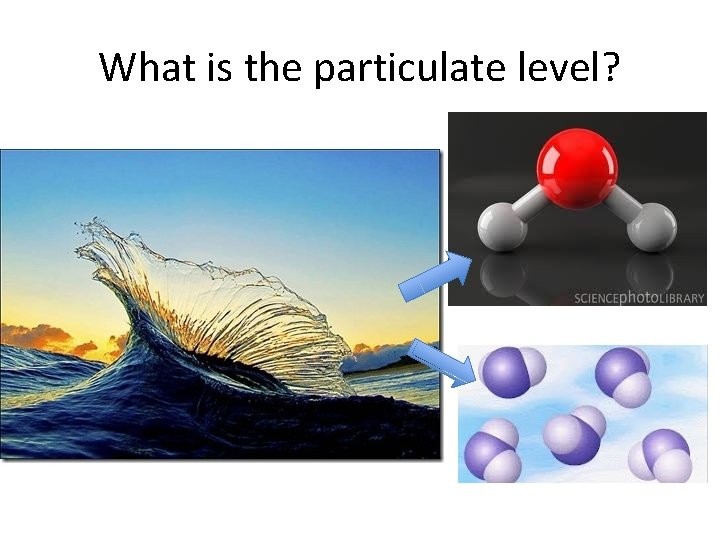What is the particulate level?What is matter is made of?Atoms • Atom: is the basic unit of any element. Atoms have space and mass and make up all forms of matter. • Element: is matter made up of only one kind of atom: Which is an element and which isn’t? A B C DHow many kinds of elements OR types of atoms are there? How do you know?How can we talk about matter?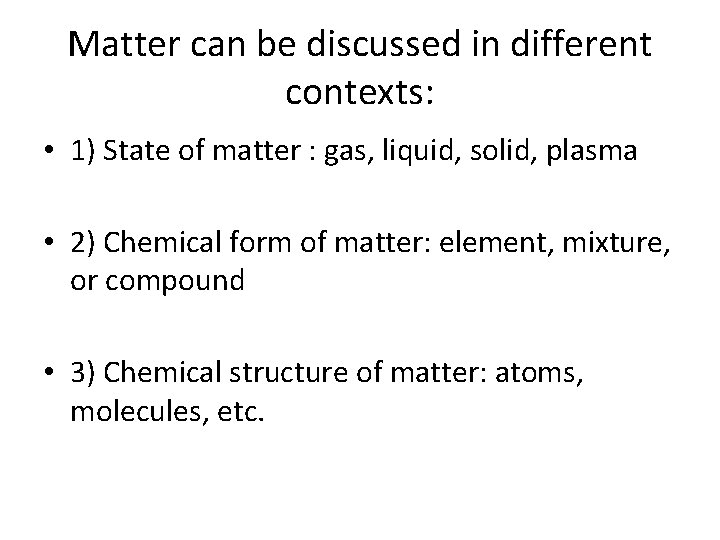Matter can be discussed in different contexts: • 1) State of matter : gas, liquid, solid, plasma • 2) Chemical form of matter: element, mixture, or compound • 3) Chemical structure of matter: atoms, molecules, etc.Use of the word “substance” in chemistry implies that it is “pure” • (Pure) substance: any sample of matter (solid, liquid, or gas) that has a definite and constant composition AND distinct chemical properties (behavior). • Examples: diamond, water, table salt, baking soda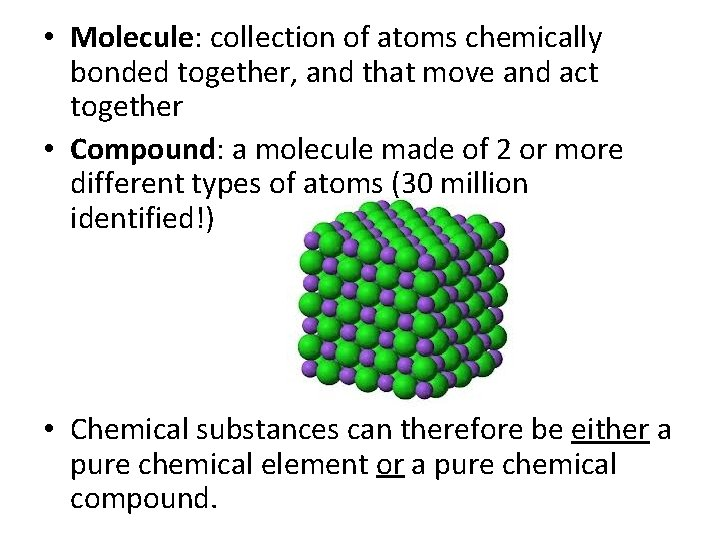• Molecule: collection of atoms chemically bonded together, and that move and act together • Compound: a molecule made of 2 or more different types of atoms (30 million identified!) • Chemical substances can therefore be either a pure chemical element or a pure chemical compound.Place the correct word by each letter: atom, compound, element or molecule (more than one label may apply) A. B. C. D. How many types atoms are in each substance?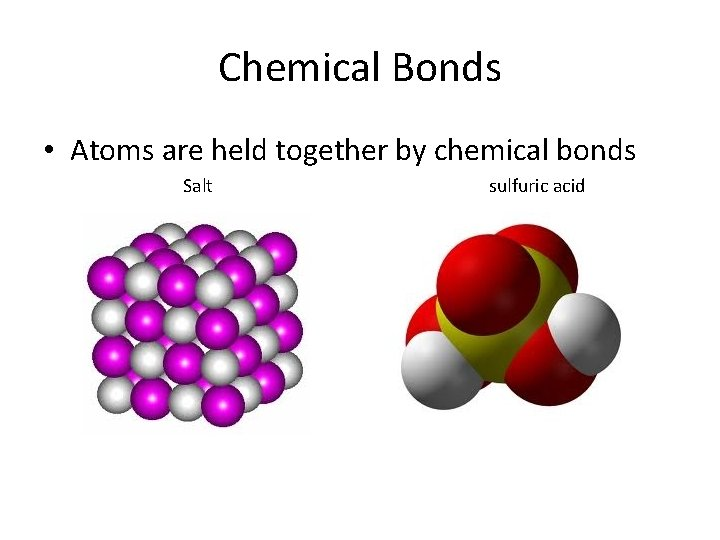Chemical Bonds • Atoms are held together by chemical bonds Salt sulfuric acidWarm Up: Apply as many of the following terms to each diagram: Terms: element, compound, molecule, atom(s)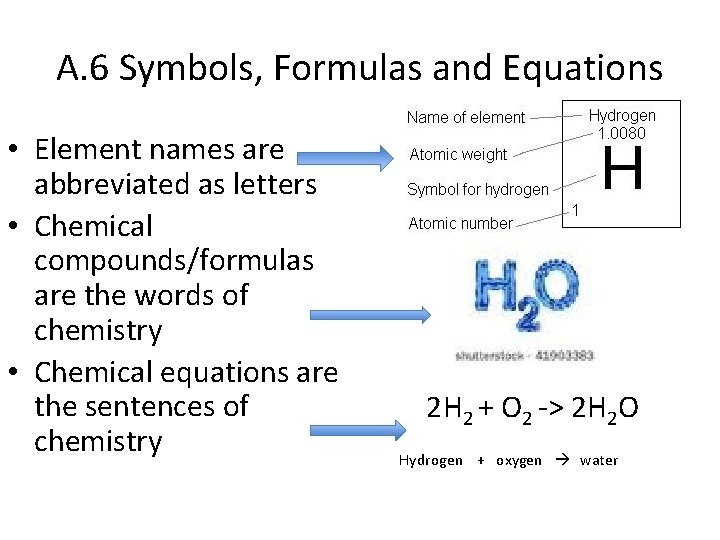A. 6 Symbols, Formulas and Equations • Element names are abbreviated as letters • Chemical compounds/formulas are the words of chemistry • Chemical equations are the sentences of chemistry 2 H 2 + O 2 -> 2 H 2 O Hydrogen + oxygen water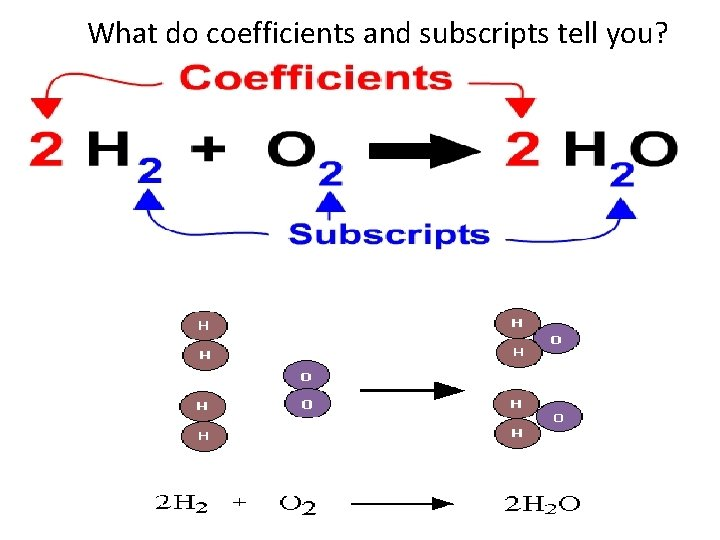What do coefficients and subscripts tell you?How many atoms total do the following formulas represent? Examples: A. H 2 B. 2 H 2 C. H 2 O 2 D. 2 H 2 O 2 • What elements are in each substance? • What is the difference between A and B? Draw a model to show the difference.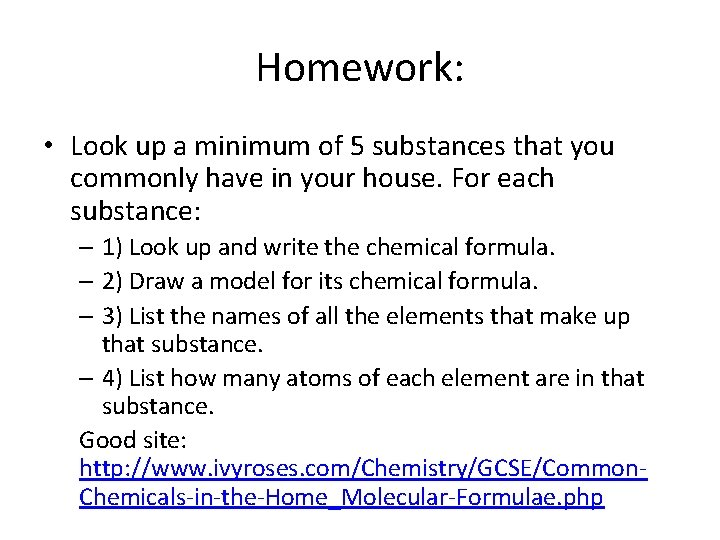Homework: • Look up a minimum of 5 substances that you commonly have in your house. For each substance: – 1) Look up and write the chemical formula. – 2) Draw a model for its chemical formula. – 3) List the names of all the elements that make up that substance. – 4) List how many atoms of each element are in that substance. Good site: http: //www. ivyroses. com/Chemistry/GCSE/Common. Chemicals-in-the-Home_Molecular-Formulae. php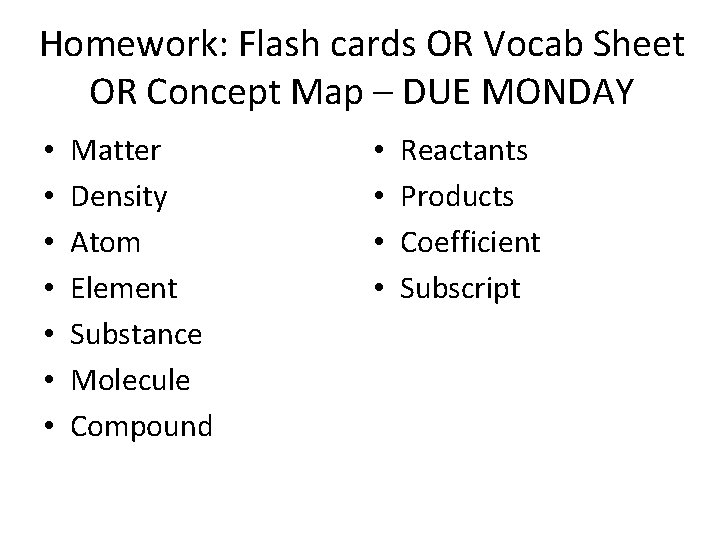Homework: Flash cards OR Vocab Sheet OR Concept Map – DUE MONDAY • • Matter Density Atom Element Substance Molecule Compound • • Reactants Products Coefficient Subscript